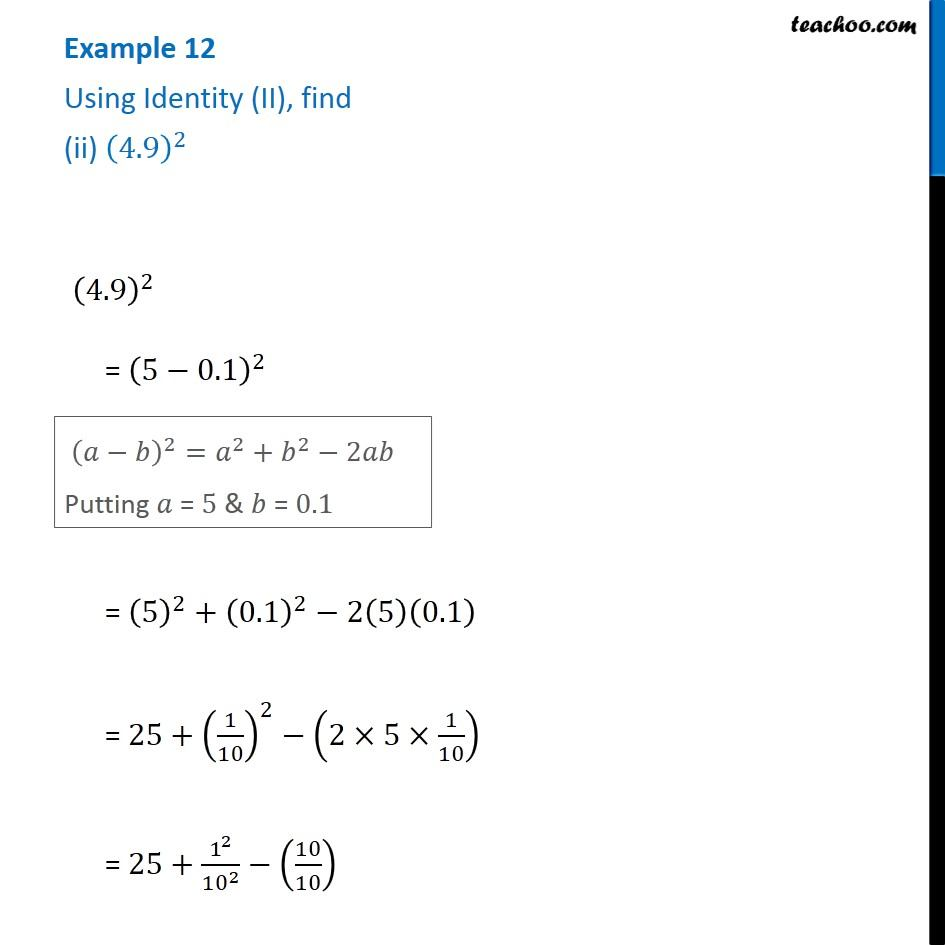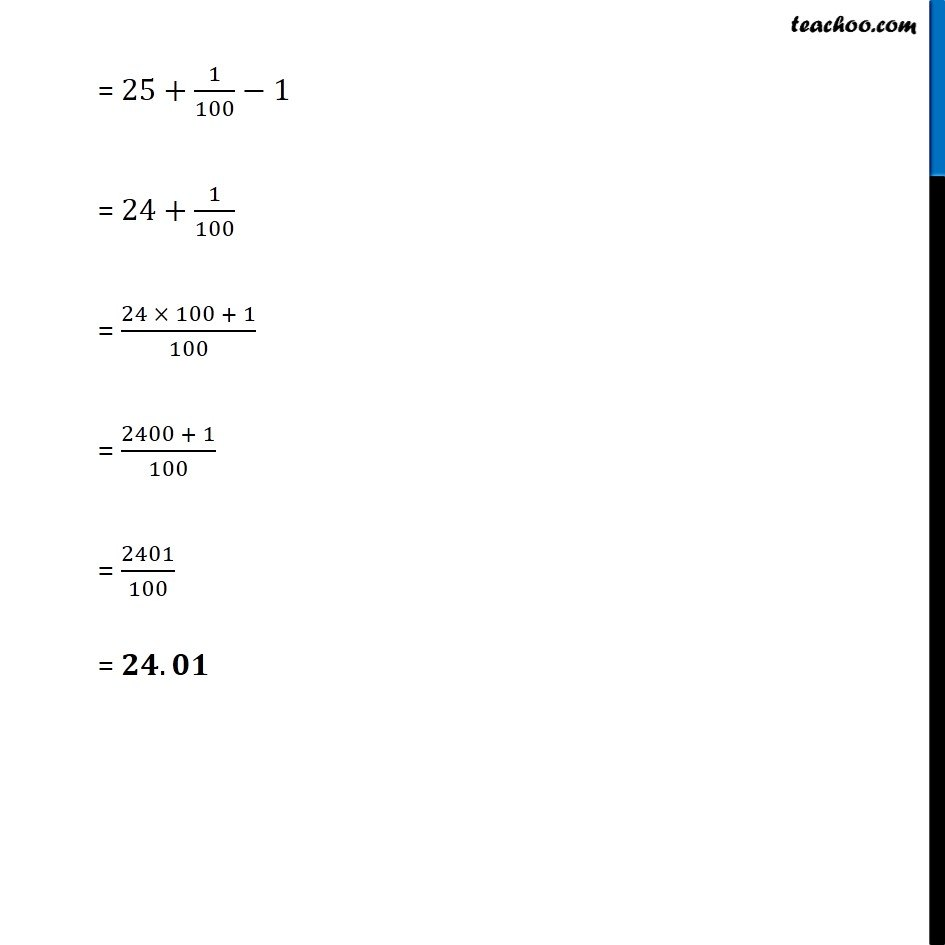Examples

Chapter 8 Class 8 Algebraic Expressions and Identities
Serial order wiseLearn in your speed, with individual attention - Teachoo Maths 1-on-1 Class

### Transcript

Example 12 Using Identity (II), find (ii) (4.9)^2 (4.9)^2 = (5−0.1)^2 (𝑎−𝑏)^2=𝑎^2+𝑏^2−2𝑎𝑏 Putting 𝑎 = 5 & 𝑏 = 0.1 = (5)^2+(0.1)^2−2(5)(0.1) = 25+(1/10)^2−(2×5×1/10) = 25+1^2/〖10〗^2 −(10/10) = 25+1/100−1 = 24+1/100 = (24 × 100 + 1)/100 = (2400 + 1)/100 = 2401/100 = 𝟐𝟒.𝟎𝟏